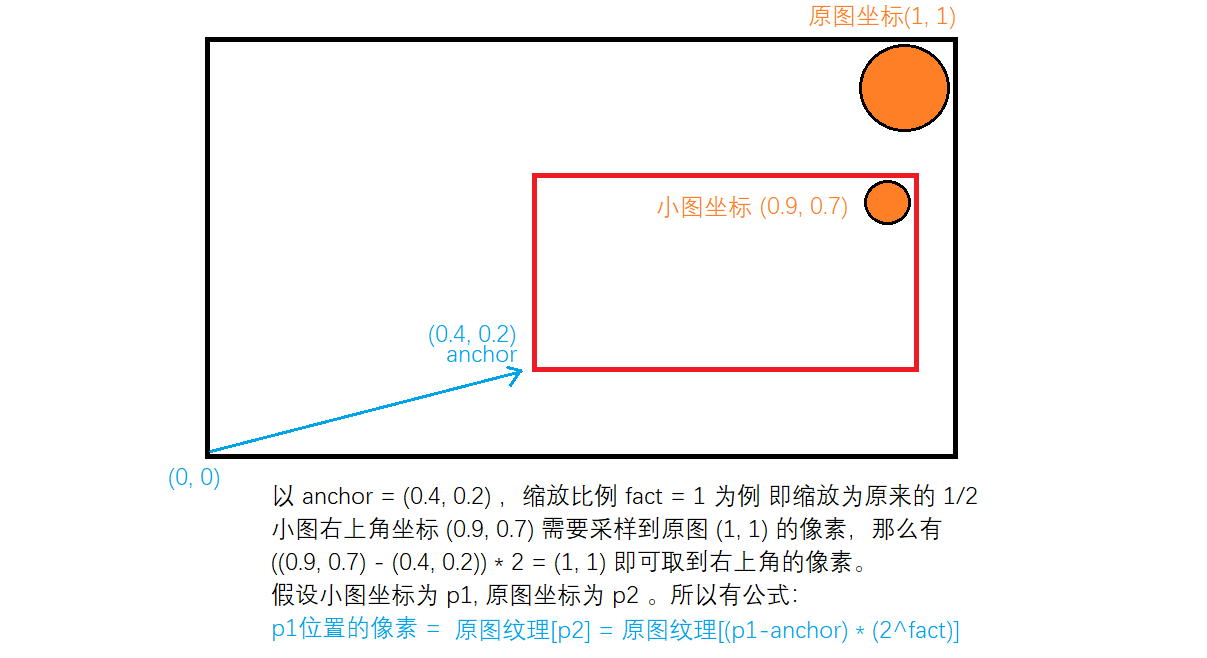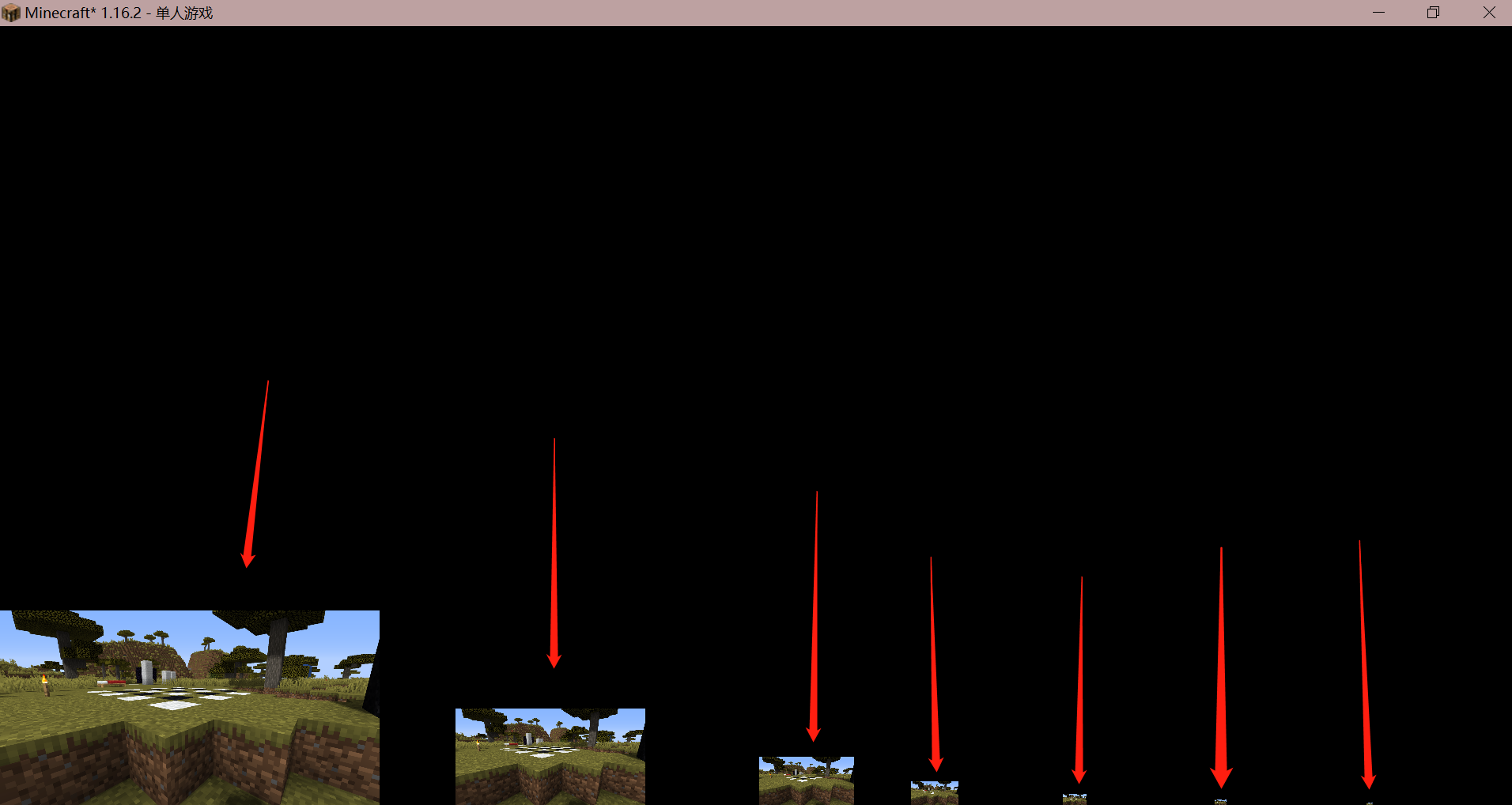# 模糊的拆分

## 原理（注：其实这么理解是有点问题的，真要证明害得看数学。其实本文的模糊并未使用高斯分布函数作为权重。。## 渲染流程

gl_FragData = getBloomOriginColor(color);


/* DRAWBUFFERS: 01 */


1. composite1.fsh 负责对1号缓冲区中的高亮原图 O 做横向的模糊，得到图像 A 并且写入1号缓冲区
2. composite1.fsh 负责对1号缓冲区中的 A 图像做纵向的模糊，得到图像 B 并且写入1号缓冲区
3. final.fsh 中负责对 0 和 1 号缓冲区中的图像做叠加，并且输出

## 代码#version 120

varying vec4 texcoord;

void main() {
gl_Position = ftransform();
texcoord = gl_TextureMatrix * gl_MultiTexCoord0;
}


1. 对 1 号缓冲区的图像进行一次横向的模糊并将结果写入 1 号缓冲区
2. 将 0 号缓冲区的原图继续传递（写）下去

#version 120

uniform sampler2D colortex0;
uniform sampler2D colortex1;

uniform float viewWidth;
uniform float viewHeight;

varying vec4 texcoord;

/* DRAWBUFFERS: 01 */
void main() {
// 传递基色
vec4 color = texture2D(colortex0, texcoord.st);
gl_FragData = color;

// 横向模糊
vec3 sum = texture2D(colortex1, texcoord.st).rgb;
vec2 offset = vec2(i/viewWidth, 0);
sum += texture2D(colortex1, texcoord.st+offset).rgb;
sum += texture2D(colortex1, texcoord.st-offset).rgb;
}
gl_FragData = vec4(sum, 1.0);
}


#version 120

uniform sampler2D colortex0;
uniform sampler2D colortex1;

uniform float viewWidth;
uniform float viewHeight;

varying vec4 texcoord;

/* DRAWBUFFERS: 01 */
void main() {
// 传递基色
vec4 color = texture2D(colortex0, texcoord.st);
gl_FragData = color;

// 横向模糊
vec3 sum = texture2D(colortex1, texcoord.st).rgb;
vec2 offset = vec2(0, i/viewHeight);
sum += texture2D(colortex1, texcoord.st+offset).rgb;
sum += texture2D(colortex1, texcoord.st-offset).rgb;
}
gl_FragData = vec4(sum, 1.0);
}#version 120

uniform sampler2D colortex0;
uniform sampler2D colortex1;

varying vec4 texcoord;

void main() {
vec4 color = texture2D(colortex0, texcoord.st);
vec4 bloom = texture2D(colortex1, texcoord.st);

color.rgb += bloom.rgb;

gl_FragData = color;
}（注 左边的数字是帧数的整数部分 而 / 右边大概是小数部分？）：# 对低分辨率纹理进行模糊## 采样低分辨率图像

vec4 color = texture2D(texture, texcoord.st);

// 中间是 composite.fsh 的其他代码

vec4 bloom = color;
gl_FragData = bloom;


#version 120

varying vec4 texcoord;

void main() {
gl_Position = ftransform();
texcoord = gl_TextureMatrix * gl_MultiTexCoord0;
}


#version 120

uniform sampler2D colortex0;
uniform sampler2D colortex1;
uniform sampler2D colortex2;

uniform float viewWidth;
uniform float viewHeight;

varying vec4 texcoord;

/*
*  @function getScale : 得到缩放采样后的图像
*  @param src         : 纹理源
*  @param pos         : 屏幕坐标
*  @param anchor      : 缩放后图像存储位置 -- 图片左下角坐标
*  @param fact        : 缩放比例为 2^fact 倍 -- 0为不缩放
*  @return            : 缩放后pos位置的像素值
*/
vec4 getScale(sampler2D src, vec2 pos, vec2 anchor, int fact) {
vec2 newCoord = (pos - anchor) * pow(2, fact);
if(newCoord.x<0 || newCoord.x>1 || newCoord.y<0 || newCoord.y>1) {
return vec4(0, 0, 0, 1);
}
return texture2D(src, newCoord);
}

/* DRAWBUFFERS: 01 */
void main() {
// 传递基色
vec4 color = texture2D(colortex0, texcoord.st);
gl_FragData = color;

// 计算不同分辨率的亮色纹理
vec4 bloom = vec4(0);
bloom += getScale(colortex1, texcoord.st, vec2(0.0, 0), 2);
bloom += getScale(colortex1, texcoord.st, vec2(0.3, 0), 3);
bloom += getScale(colortex1, texcoord.st, vec2(0.5, 0), 4);
bloom += getScale(colortex1, texcoord.st, vec2(0.6, 0), 5);
bloom += getScale(colortex1, texcoord.st, vec2(0.7, 0), 6);
bloom += getScale(colortex1, texcoord.st, vec2(0.8, 0), 7);
bloom += getScale(colortex1, texcoord.st, vec2(0.9, 0), 8);
gl_FragData = bloom;
}vec4 color = texture2D(colortex1, texcoord.st);
gl_FragData = color;/*
*  @function getScaleInverse : 得到缩放采样后的图像
*  @param src                : 源纹理
*  @param pos                : 屏幕坐标 xy 轴
*  @param anchor             : 缩放图像存储位置 -- 左下角坐标
*  @param fact               : 缩放比例为 2^fact 倍
*  @return                   : 从缩放中还原的像素值
*/
vec4 getScaleInverse(sampler2D src, vec2 pos, vec2 anchor, int fact) {
return texture2D(src, pos/pow(2, fact)+anchor);
}


gl_FragData = getScaleInverse(colortex1, texcoord.st, vec2(0.5, 0), 4);## 模糊处理

1. 获取下采样的小图
2. 将小图还原到屏幕上

/*
*  @function getScale : 得到缩放采样后的图像
*  @param src         : 纹理源
*  @param pos         : 屏幕坐标
*  @param anchor      : 缩放后图像存储位置 -- 图片左下角坐标
*  @param fact        : 缩放比例为 2^fact 倍 -- 0为不缩放
*  @return            : 缩放后pos位置的像素值
*/
vec4 getScale(sampler2D src, vec2 pos, vec2 anchor, int fact) {
vec2 newCoord = (pos - anchor) * pow(2, fact);
if(newCoord.x<0 || newCoord.x>1 || newCoord.y<0 || newCoord.y>1) {
return vec4(0, 0, 0, 1);
}
// 模糊
vec4 sum = texture2D(src, newCoord);
int radius = 3;	// 半径
// 计算权重
float weight = 1.0f - length(vec2(i, j)) / 3.5;
// 计算偏移
vec2 offset = vec2(float(i)/viewWidth, float(j)/viewHeight) * pow(2, fact);
// 左上左下右上右下采样4次
sum.rgb += texture2D(src, newCoord+offset).rgb * weight;
offset = vec2(-float(i)/viewWidth, float(j)/viewHeight) * pow(2, fact);
sum.rgb += texture2D(src, newCoord+offset).rgb * weight;
offset = vec2(float(i)/viewWidth, -float(j)/viewHeight) * pow(2, fact);
sum.rgb += texture2D(src, newCoord+offset).rgb * weight;
offset = vec2(-float(i)/viewWidth, -float(j)/viewHeight) * pow(2, fact);
sum.rgb += texture2D(src, newCoord+offset).rgb * weight;
}
}
}


vec4 color = texture2D(colortex0, texcoord.st);

vec4 bloom = vec4(vec3(0), 1);
bloom.rgb += getScaleInverse(colortex1, texcoord.st, vec2(0.0, 0), 2).rgb * pow(7, 0.25);
bloom.rgb += getScaleInverse(colortex1, texcoord.st, vec2(0.3, 0), 3).rgb * pow(6, 0.25);
bloom.rgb += getScaleInverse(colortex1, texcoord.st, vec2(0.5, 0), 4).rgb * pow(5, 0.25);
bloom.rgb += getScaleInverse(colortex1, texcoord.st, vec2(0.6, 0), 5).rgb * pow(4, 0.25);
bloom.rgb += getScaleInverse(colortex1, texcoord.st, vec2(0.7, 0), 6).rgb * pow(3, 0.25);
bloom.rgb += getScaleInverse(colortex1, texcoord.st, vec2(0.8, 0), 7).rgb * pow(2, 0.25);
bloom.rgb += getScaleInverse(colortex1, texcoord.st, vec2(0.9, 0), 8).rgb * pow(1, 0.25);
bloom.rgb = pow(bloom.rgb, vec3(1/2.2));

color.rgb += bloom.rgb * 0.5;
gl_FragData = color;## 分辨光源方块1. 输出方块原颜色 * 方块纹理
2. 输出光照，将光照纹理乘以输出图像
3. 输出顶点属性 mc_Entity 表示方块ID 到 2 号颜色缓冲区

gbuffer_terrain.vsh

#version 120

varying vec4 texcoord;
varying vec4 lightMapCoord;
varying vec3 color;
varying float blockId;

attribute vec4 mc_Entity;

void main() {
gl_Position = ftransform();
color = gl_Color.rgb;   // 方块原色
texcoord = gl_TextureMatrix * gl_MultiTexCoord0; // 方块纹理坐标
lightMapCoord = gl_TextureMatrix * gl_MultiTexCoord1;    // 光照纹理坐标
blockId = mc_Entity.x;  // 方块id
}


gbuffer_terrain.fsh：

#version 120

// 声明2号颜色缓冲区为 R32F 格式 只有x通道可用 传递方块id
const int R32F = 114;
const int colortex2Format = R32F;

uniform sampler2D texture;
uniform sampler2D lightmap;

varying vec4 texcoord;
varying vec4 lightMapCoord;
varying vec3 color;
varying float blockId;

/* DRAWBUFFERS:02 */
void main() {
// 纹理 * 颜色
vec4 blockColor = texture2D(texture, texcoord.st);
blockColor.rgb *= color;

// 光照
vec3 light = texture2D(lightmap, lightMapCoord.st).rgb;
blockColor.rgb *= light;

gl_FragData = blockColor;
gl_FragData = vec4(blockId);
}block.10089 = glowstone redstone_lamp:lit=true furnace:lit=true blast_furnace:lit=true smoker:lit=true jack_o_lantern beacon campfire lantern shroomlight soul_lantern soul_torch soul_fire soul_campfire:lit=true fire flowing_lava lava
block.10090 = torch wall_torch redstone_torch:lit=true redstone_wall_torch:lit=true


block.自定义的id = 方块名称1 方块名称2 ....

1. 如果是荧石等发光方块，我们直接在原图上乘一个颜色并且输出
2. 如果是火把，我们提取亮色之后，根据亮度作为权值输出，即亮的像素权值高
3. 如果是其他方块，泛光的颜色变为原来的 1/10
vec4 color = texture2D(texture, texcoord.st);

// 其他的代码

vec4 bloom = color;
float id = texture2D(colortex2, texcoord.st).x;
// 发光方块
if(id==10089) {
bloom.rgb *= vec3(0.7, 0.4, 0.2);
}
// 火把
else if(id==10090) {
float brightness = dot(bloom.rgb, vec3(0.2, 0.7, 0.1));
if(brightness < 0.5) {
bloom.rgb = vec3(0);
}
bloom.rgb *= (brightness-0.5)*2;
}
// 其他
else {
bloom.rgb *= 0.1;
}

gl_FragData = color;
gl_FragData = bloom;## 使用mipmapconst bool gdepthMipmapEnabled = true;if(newCoord.x<0 || newCoord.x>1 || newCoord.y<0 || newCoord.y>1) {
return vec4(0, 0, 0, 1);
}


float padding = 0.02 * pow(2, fact);
return vec4(0, 0, 0, 1);
}# 小结

10-038504-04
08-31
09-16164
09-14214
09-05
09-1989